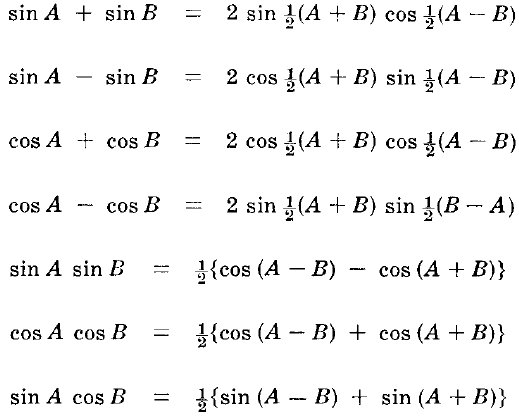# Trigonometric Functions Formulas - Single,Half,Double,Multiple Angles# Basic Trigonometric Functions

## Definition of Trigonometric Functions For a Right Angle

Triangle ABC bas a right angle (9O°) at C and sides of length a, b, c. The trigonometric functions of angle A are defined as follows.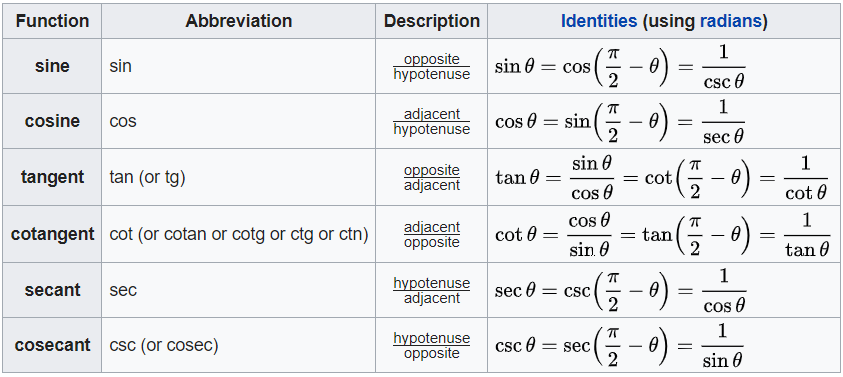# Extensions To Angles Which May be Greater Than 90°

Consider an xy coordinate system . A point P in the xy plane has coordinates (x,y) where x is eonsidered as positive along OX and negative along OX´ while y is positive along OY and negative along OY´ .

The distance from origin 0 to point P is positive and denoted by r = √x2 + y2

The angle A described counterclockwise from OX is considered positive. If it is described dockhse from

OX it is considered negative. We cal1 X´ OX and Y´ OY the x and y axis respectively.

The various quadrants are denoted by 1, II, III and IV called the first, second, third and fourth quadrants respectively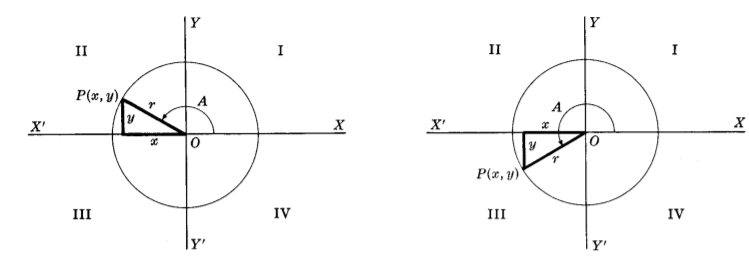For an angle A in any quadrant the trigonometric functions of A are defined as follows.

sin A = y/r

cos A = x/r

tan A = y/x

cet A = x/y

sec A = r/x

csc A = r/y

## Signs And Variations Of Trigonometric Functions

tan A = Sin A / Cos A

Cot A = 1 / tan A = Cos A / Sin A

Sec A = 1 / Cos A

Csc A = 1 / Sin A

Sin2 A + Cos2 A = 1

Sec2 A - tan2 A = 1

Csc2 A - Cot2 A = 1

## Exact Values For Trigonometric Functions Of Various Angles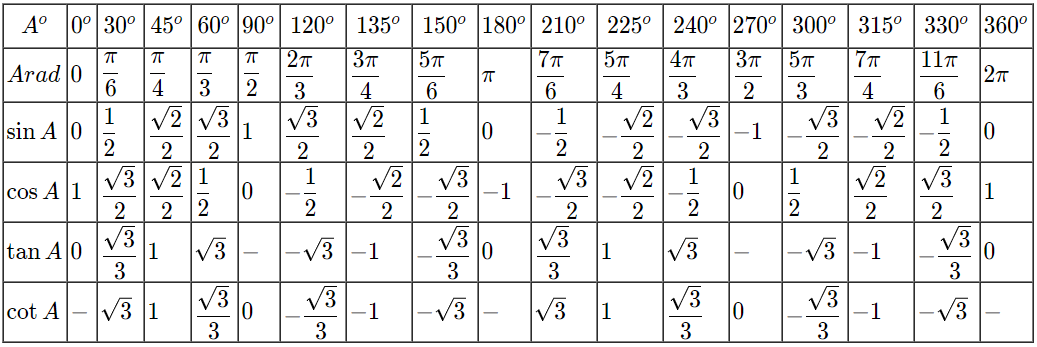## Functions Of Negative Angles

Sin (-A) = - Sin A

Cos (-A) = Cos A

tan (-A) = - tan A

Csc (-A) = - Csc A

Sec (-A) = Sec A

Cot (-A) = - Cot A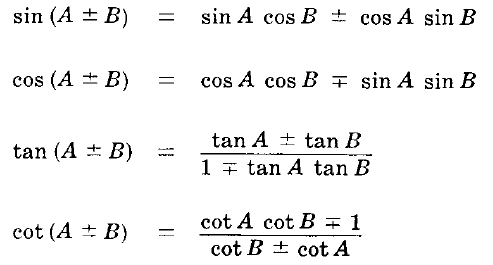## Functions Of Angles in All Quadrants in Terms Of Those in Quadrant I## Relationships Amoung Functions Of Angles in Quadrant I## Double Angle Formulas

Sin 2A = 2 Sin A Cos A

Cos 2A = Cos2A - Sin2A = 1 - 2 Sin2A = 2 Cos2A - 1

tan 2A = 2 tan A / 1 - tan2A

## Half Angle Formulas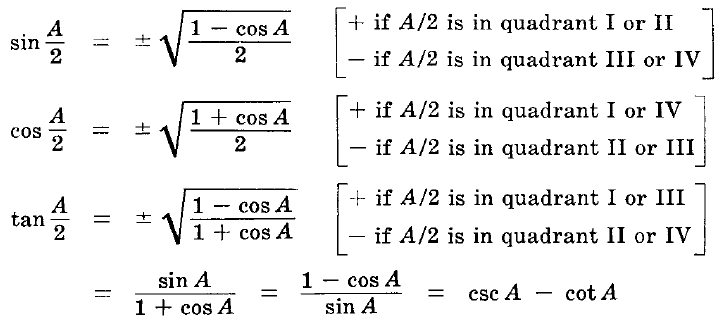## Multiple Angle Formulas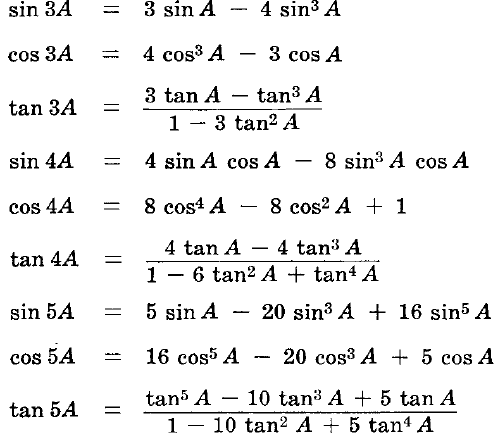## Powers of Trigonometric Functions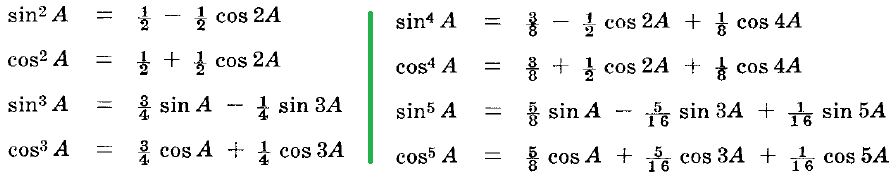## Sum, Difference and Product Of Trigonometric Functions